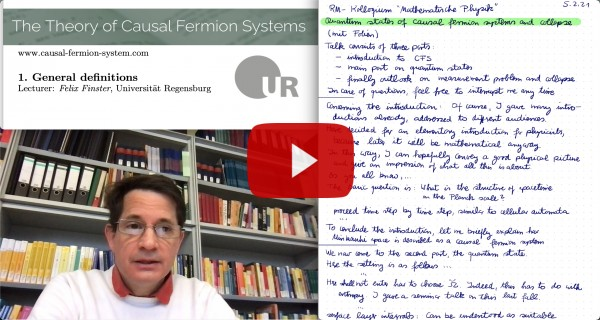# The Theory of Causal Fermion Systems

## The Fermionic Projector## The Kernel of the Fermionic Projector

The kernel of the fermionic projector defined by

$P(x,y) = \pi_x \,y|_{S_y} \::\: S_y \longrightarrow S_x$

is a mapping from one spin space to another. It gives relations between different spacetime points $x, y \in M$. It can be decomposed into physical wave functions. Indeed, choosing an orthonormal basis $(e_i)$ of $\H$,

$P(x,y) \, \phi = \pi_x y|_{S_y} \,\phi = \sum_i \pi_x e_i \: \la e_i |\; y\,\phi \ra_\H = -\sum_i \psi^{e_i}(x) \: \Sl \psi^{e_i}(y) |\; \phi \Sr_y \:.$

The kernel of the fermionic projector plays a central role for several reasons:

• The Lagrangian and the constraints can be expressed in terms of $P(x,y)$. This can be understood from the fact that the operators $xy : \H \rightarrow \H$ and $\pi_y xy|_{S_y} : S_y \rightarrow S_y$ have the same eigenvalues and algebraic multiplicities, up to zeros in the spectrum. The latter operator can be expressed in terms of the kernel of the fermionic projector by $\pi_y \, x y|_{S_y} = \pi_y \, x\, \pi_x \, y|_{S_y} = P(y,x)\, P(x,y) \::\: S_y \rightarrow S_y \:.$ The combination $P(y,x)\, P(y,x)$ is also referred to as the closed chain.
• Being a mapping from one spin space to another, it gives relations between different spacetime points. In this way, it carries all the information on the causal and geometric structure of spacetime.
• Being composed of all the physical wave functions, it carries all the information on the distribution of matter in spacetime.

## The Fermionic Projector as an Operator on Wave Functions

The kernel of the fermionic projector can be taken as the integral kernel of a corresponding integral operator $P$,

$(P \psi)(x) := \int_M P(x,y)\: \psi(y)\: d\rho(y) \:,$

referred to as the fermionic projector. Here $\psi$ is a wave function (i.e. a mapping from $M$ to $\H$ with the property that $\psi(x) \in S_x$ for all $x \in M$; in other words, $\psi$ is a section of the topological spinor bundle). The fermionic projector is a symmetric operator if the wave functions are endowed with the indefinite inner product

$\displaystyle \bra \psi | \phi \ket = \int_M \Sl \psi(x) | \phi(x) \Sr_x \: d\rho(x) \:.$

This indefinite inner product can be given the structure of a Krein space with the topology induced by the scalar product

$\la\!\la \psi | \phi \ra\!\ra = \int_M \la \psi(x) |\, |x|\, \phi(x) \ra_\H \:d\rho(x) \:.$

(where $|x|$ is the absolute value of the operator $x$). The fermionic projector is an in general unbounded, densely defined linear operator on this Krein space. The operator $(-P)$ is positive in the sense that for all $\psi$ in the domain,

$\bra \psi | (-P) \psi \ket \geq 0 \:.$